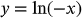1
36
views
61d
Problem

Problem 61d

Chapter 1: Functions and ModelsTextbook ExpertVerified Tutor
18 Oct 2021

Given information

The given equation is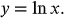Step-by-step explanation

Step 1.

Transformation rule: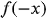is the graph of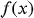reflected about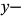axis.

Draw the graphs of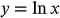and its reflection aboutaxis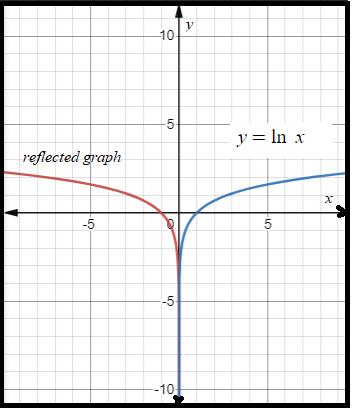The equation of the reflected graph is